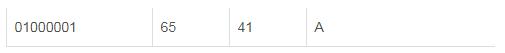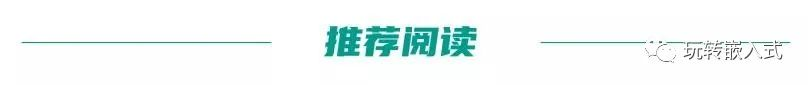• 编程时经常用到进制转换、字符转换。比如软件界面输入的数字字符串，如何将字符串...01字符串转十六进制代码实现：voidStrToHex(char*pbDest,char*pbSrc,intnLen){charh1,h2;chars1,s2;inti;for(i=0;i{h1=pbSrc[2*i...编程时经常用到进制转换、字符转换。比如软件界面输入的数字字符串，如何将字符串处理成数字呢？和大家分享一下。01字符串转十六进制代码实现：void StrToHex(char *pbDest, char *pbSrc, int nLen){  char h1,h2;  char s1,s2;  int i;    for (i=0; i    {        h1 = pbSrc[2*i];        h2 = pbSrc[2*i+1];        s1 = toupper(h1) - 0x30; //toupper 转换为大写字母        if (s1 > 9)            s1 -= 7;        s2 = toupper(h2) - 0x30;        if (s2 > 9)            s2 -= 7;        pbDest[i] = s1*16 + s2;    }}02十六进制转字符串代码实现：void HexToStr(char *pszDest, char *pbSrc, int nLen){    char    ddl, ddh;    for (int i = 0; i     {        ddh = 48 + pbSrc[i] / 16;        ddl = 48 + pbSrc[i] % 16;        if (ddh > 57) ddh = ddh + 7;        if (ddl > 57) ddl = ddl + 7;        pszDest[i * 2] = ddh;        pszDest[i * 2 + 1] = ddl;    }    pszDest[nLen * 2] = '\0';}或者：u16 Hex2StringArray (u8 *pSrc,  u16 SrcLen, u8 *pObj){    u16 i=0;    for(i=0;    i    {        sprintf((char *)(pObj + i * 2), "%02X", *(pSrc + i));    }    *(pObj + i * 2) = '\0';    return  (i * 2);}效果：十六进制：0x13 0xAA 0x02转为字符串：13AAA203字符串转十进制代码实现：第一种，如果带负号 这个就是atoi函数的实现：int my_atoi(const char *str){    int value = 0;    int flag = 1; //判断符号    while (*str == ' ')  //跳过字符串前面的空格    {        str++;    }    if (*str == '-')  //第一个字符若是‘-’，说明可能是负数    {        flag = 0;        str++;    }    else if (*str == '+') //第一个字符若是‘+’，说明可能是正数    {        flag = 1;        str++;    }//第一个字符若不是‘+’‘-’也不是数字字符，直接返回0    else if (*str >= '9' || *str <= '0')     {        return 0;        }    //当遇到非数字字符或遇到‘\0’时，结束转化    while (*str != '\0' && *str <= '9' && *str >= '0')    {        value = value * 10 + *str - '0'; //将数字字符转为对应的整形数        str++;    }    if (flag == 0) //负数的情况    {        value = -value;    }    return value;}效果：字符串：-123 转为 -123第二种，如果不带负号void StrtoDec(uint32_t *pbDest, char *pbSrc, int nLen){    int i;    int tmp=0;    if(nLen > 10)        *pbDest = 0;    tmp = 1;    *pbDest = 0;    for (i=nLen-1; i>=0; i--)    {        *pbDest += tmp*(*(pbSrc+i)-'0');        tmp = tmp*10;    }}效果：字符串：123转为 123第三种：包含转为浮点数//m^n函数//返回值:m^n次方.u32 NMEA_Pow(u8 m,u8 n){    u32 result=1;        while(n--)result*=m;        return result;}//str转换为数字,以','或者'*'结束//buf:数字存储区//dx:小数点位数,返回给调用函数//返回值:转换后的数值int NMEA_Str2num(u8 *buf,u8*dx){    u8 *p=buf;    u32 ires=0,fres=0;    u8 ilen=0,flen=0,i;    u8 mask=0;    int res;    while(1) //得到整数和小数的长度    {        if(*p=='-'){mask|=0X02;p++;}//是负数        if(*p==','||(*p=='*'))break;//遇到结束了        if(*p=='.'){mask|=0X01;p++;}//遇到小数点了        else if(*p>'9'||(*p<'0'))   //有非法字符        {               ilen=0;            flen=0;            break;        }           if(mask&0X01)flen++;        else ilen++;        p++;    }    if(mask&0X02)buf++; //去掉负号    for(i=0;i    {          ires+=NMEA_Pow(10,ilen-1-i)*(buf[i]-'0');    }    if(flen>5)flen=5;   //最多取5位小数    *dx=flen;           //小数点位数    for(i=0;i    {          fres+=NMEA_Pow(10,flen-1-i)*(buf[ilen+1+i]-'0');    }     res=ires*NMEA_Pow(10,flen)+fres;    if(mask&0X02)res=-res;             return res;} 效果：字符串：”123.456” 先转为 123456，然后除以1000得到123.45604十进制转字符串代码实现：如果只是单个十进制转字符串，使用sprintf函数就可以了。如果是十进制数组：u16 DectoStr (u8 *pSrc, u16 SrcLen, u8 *pObj){    u16 i=0;    for(i=0;    i    {        sprintf((char *)(pObj + i * 2), "%02d", *(pSrc + i));    }    *(pObj + i * 2) = '\0';    return  (i * 2);}效果：十进制数组13 14转为字符串“1314”05u8、u32转换举个栗子：ASCII码里这里写图片描述 字符‘A’ ， 一个字节8bit ，即u8 十六进制为 0x41 二进制为 0100 0001而对应的十进制为 65 整型65，4个字节32bit，即u32 十六进制为 0x41 二进制为 0000 0000 0000 0000 0000 0000 0100 0001将u32数转换成u8数组注意：这里是字符数组，不是字符串字符串是以空字符(\0)结尾的char数组void U32ToU8Array(uint8_t *buf, uint32_t u32Value){    buf = ((u32Value >> 24) & 0xFF);    buf = ((u32Value >> 16) & 0xFF);    buf = ((u32Value >> 8) & 0xFF);    buf = (u32Value & 0xFF);}效果：整型 50 转字符数组 {‘\0’,’\0’,’\0’,’2’}u8数组转u32void U8ArrayToU32(uint8_t *buf, uint32_t *u32Value){    *u32Value = (buf <<24) + (buf <<16) + (buf <<8) + (buf <<0);}效果：字符数组 {‘\0’,’\0’,’\0’,’2’}转为整型 5006大小端(高低位)转换STM32 默认是小端模式的，那么该如何转为大端？//为大端：pPack = (u8)((len >> 8) & 0xFF);pPack = (u8)(len & 0xFF);//为小端：pPack = (u8)(len & 0xFF);pPack =  (u8)((len >> 8) & 0xFF);效果：len为数据类型为 u16(short)，比如 0x11 0x22，转为u8(usigned char)数组。大端为：pPack (0x11 ) pPack (0x22) 小端为：pPack (0x22) pPack (0x11)【1】C语言，动态展示经典排序算法【2】单片机常用的14个C语言算法，看过的都成了大神！【3】插入排序：最直观的排序算法【4】冒泡排序，经典的排序算法【5】卡尔曼滤波算法，啥原理？【6】C语言编程建议和技巧，同样适用于单片机的学习展开全文• #二进制转化成十六进制print("请输入一个二进制数\n")SUM1=[]SUM2=[]A=0B=0int1=[]#整数部分存放处float1=[]#小数部分存放处a1=int(input("请输入二进制的整数部分"))print("a1=",a1)#整数部分a2=input("请输入二...
#二进制转化成十六进制print("请输入一个二进制数\n")SUM1=[]SUM2=[]A=0B=0int1=[]#整数部分存放处float1=[]#小数部分存放处a1=int(input("请输入二进制的整数部分"))print("a1=",a1)#整数部分a2=input("请输入二进制的小数部分")print("a2=",a2)for x in a2:float1.append(int(x))while(a1&gt=1):int1.append(a1%10)a1=a1//10print("int1=",int1)print("float1=",float1)def sumint(v):j=0summ=0for i in v:summ=summ+i*(2**j)j=j+1if summ==10:summ="A"if summ==11:summ="B"if summ==12:summ="C"if summ==13:summ=""if summ==14:summ="E"if summ==15:summ="F"return summ#输入四位二进制，输出一个十六进制int2=[]while(A&ltlen(int1)):i=int1[A]int2.append(i)if len(int2)==4:print(int2)SUM1.append(sumint(int2))int2=[]elif A+1==len(int1):print(int2)SUM1.append(sumint(int2))else:passA=A+1#整数部分float2=[]while B&ltlen(float1):I=float1[B]float2.insert(0,I)if len(float2)==4:SUM2.append(sumint(float2))float2=[]elif B+1==len(float1):if len(float2)==1:SUM2.append(8)if len(float2)==2:float2.insert(0,0)float2.insert(0,0)SUM2.append(sumint(float2))if len(float2)==3:float2.insert(0,0)SUM2.append(sumint(float2))B=B+1#小数部分print(SUM1)SUM1.reverse()print("最终求得的十六进制是",end=" ")for i in SUM1:print(i,end="")print(".",end="")for ii in SUM2:print(ii,end="")
展开全文• 进制转换C++代码#include#include#include#includeusing namespace std;void MainMenu(){system("cls");for(int i=0;i<24;i++) cout<printf("\n1. 10->2\n2. 2->10\n3. 16->2\n4. 2->16\n5. 10-&...
进制转换C++代码#include#include#include#includeusing namespace std;void MainMenu(){system("cls");for(int i=0;i<24;i++) cout<printf("\n1. 10->2\n2. 2->10\n3. 16->2\n4. 2->16\n5. 10->16\n6. 16->10\n7. Exit\n");for(i=0;i<24;i++)  cout<}void mission1(){system("cls");double  x,bb;int i,aa,n,m,p,q;cout<cin>>x;aa=(int)x;if(x-aa==0){i=0;while(aa){p[i]=(int)(aa%2);aa=aa/2;i++;}cout<n=i;for(i=n-1;i>=0;i--)   cout<cout<}else{bb=x-aa;i=0;while(aa){p[i]=(int)(aa%2);aa=aa/2;i++;}n=i;i=0;while(bb){q[i]=(int)(bb*2);bb=bb*2-q[i];i++;}m=i;cout<for(i=n-1;i>=0;i--)  cout<cout<for(i=0;icout<}}void mission2(){system("cls");int i,j,n;double k,sum=0;string str;cout<cin>>str;i=0;while(in=i;//cout<if(n==str.size()){j=1;for(i=n-1;i>=0;i--){   sum+=(str[i]-'0')*j;j*=2;}cout<}else if(n{j=1;for(i=n-1;i>=0;i--){sum+=(str[i]-'0')*j;j*=2;}k=1.0/2;for(i=n+1;i{sum+=(str[i]-'0')*k;k*=1.0/2;}cout<}}void A1(char c){if(c=='0') cout<else if(c=='1')  cout<else if(c=='2')  cout<else if(c=='3')  cout<else if(c=='4')  cout<else if(c=='5')  cout<else if(c=='6')  cout<else if(c=='7')  cout<else if(c=='8')  cout<else if(c=='9')  cout<else if(c=='A')  cout<else if(c=='B')  cout<else if(c=='C')  cout<else if(c=='D')  cout<else if(c=='E')  cout<else if(c=='F')  cout<else cout<}void A(string s){int i,m,n;n=s.length();i=0;while(im=i;for(i=0;iA1(s[i]);if(m{cout<for(i=m+1;iA1(s[i]);}cout<}void mission3(){system("cls");string str;cout<cin>>str;cout<A(str);}char B2(string s){char ret;if(s=="0000")  ret='0';else if(s=="0001") ret='1';else if(s=="0010")  ret='2';else if(s=="0011")  ret='3';else if(s=="0100")  ret='4';else if(s=="0101")  ret='5';else if(s=="0110")  ret='6';else if(s=="0111")  ret='7';else if(s=="1000")  ret='8';else if(s=="1001")  ret='9';else if(s=="1010")  ret='A';else if(s=="1011")  ret='B';else if(s=="1100")  ret='C';else if(s=="1101")  ret='D';else if(s=="1110"
展开全文• 如何用c语言把十进制小数转换成二进制，八进制，十六进制。求代码？谢谢c
• 我编写了一个java程序,它应该将小数从1转换为256到十六进制,但问题是当我尝试小数超过256之后,我开始得到不正确的结果.这是我的代码：public class Conversion {public static void main(String[] args) {System.out...
我编写了一个java程序,它应该将小数从1转换为256到十六进制,但问题是当我尝试小数超过256之后,我开始得到不正确的结果.这是我的代码：public class Conversion {public static void main(String[] args) {System.out.printf("%s%14s", "Decimal", "Hexadecimal");for(int i = 1; i <= 300; i++) {System.out.printf("%7d          ", i);decimalToHex(i);System.out.println();}}private static void decimalToHex(int decimal) {int count;if(decimal >= 256) {count = 2;} else {count = 1;}for (int i = 1; i <= count; i++) {if(decimal >= 256) {returnHex(decimal / 256);decimal %= 256;}if(decimal >= 16) {returnHex(decimal / 16);decimal %= 16;}returnHex(decimal);decimal /= 16;}}private static void returnHex(int number) {switch(number) {case 15:System.out.print("F");break;case 14:System.out.print("E");break;case 13:System.out.print("D");break;case 12:System.out.print("C");break;case 11:System.out.print("B");break;case 10:System.out.print("A");break;default:System.out.printf("%d", number);break;}}}这是我得到的结果的样本：254              FE255              FF256              100257              111264              199266              1AA271              1FF272              1100273              1111注意：我刚刚开始学习java,所以如果可以,请保持简单.谢谢
展开全文• 3）将补码格式的十进制小数转换为有符号十六进制数 4）将生成的十六进制数写入.coe文件中 代码实现 % generate the random number for i = 1: 10 M(i,1)= (-1)^i *rand(1); end N = length(M); Radix = 16; Coeff...matlab
• 十进制浮点数，整数部分转换成二进制，采用除2取余法，将余数从低到高排列，即为整数的二进制数； 小数部分转换成二进制，采用乘2取整法，将取整数顺序排列，即为小数的二进制数。 小数部分乘2直到小数部分为0，或...
• 题目： 编写程序实现任意十进制正小数m转换成n进制的正小数，小数点后保留10位小数。 输入包含两个数m，n，用空格隔开。输入包含多组测试，当m，n都...第一次做笔试题，刚好抽到了与前一晚做的十进制转十六进制整数...java 字符串
• 小数的二进制转十进制 看到的都是 理论 没有代码， 今天把自己的公布下，欢迎指正（部分是借用别人的）如有疏漏，恳请指出。 #include <stdio.h> #include <string.h> #include <math.h> void ....c语言
• 代码中的进制书写形式 默认为十进制 以0开头为八进制 以0b或0B开头是二进制 ...十进制转任意进制 十进制转换为R进制数，需要将整数部分和小数部分分别转换 整数转换 用十进制的整数部分除以R，取其余数作为R进制...
• 代码由本人原创 是大一上学期的课程设计 使用C++编写 进制转化支持二进制八进制十进制十六进制的转化 并且支持小数和负数的情况 有完整人性化菜单设计 还有容错功能 需要的童鞋赶紧看看吧
• 问题分析掌握不同数制间的转换关系是解决问题的关键，这里所说的数制一般包括二进制、八进制、十六进制十进制。除了不同的数制还有下面几个必须要了解的概念。基数：在一种数制中，只能使用一组固定的数字来表示数...
• 关于本文章内容说明 文章基本只是将作者本人编写的代码进行复制粘贴，而这份代码是作者大一上学期期末作业，若有不足之处，还请...2.分离十进制数值的整数和小数部分； 3.对分离出的整数、小数部分分别做转换，将其转换c++
• //x,y为坐标 Num为想要转换的数（可以是十进制，也可以是十六进制） //len为想要转换的二进制的位数 size为字体大小 void OLED_ShowBin(u8 x,u8 y,u16 Num,u8 len,u8 size) { u16 i = 1u << len-1; //左移len...stm32 oled屏幕
• 十进制转换为二进制：整数除二取余，小数乘二取整 二进制转换为八进制，十六进制：八进制为三个一组 十六进制为四个一组 使用代码进行进制转换 类型转换 进制转换： 将int类型以不同的进制展现出来 类型转化： 将一...python
• 数据类型 1.1整型int py2中有int有范围,超出自动转为long长...十进制转二进制 print(bin(10)）十进制转八进制 print(oct(10))十进制转十六进制 print(hex(10)) 1.2布尔值bool 只有True和False. 0, '' , [] , ...
• float()函数返回一个十进制浮点型数值（小数）。 float()括号内只能是三种类型的数据： 1.二进制、八进制、十进制十六进制的整数。 2.bool（布尔值True和False）。 3.表示十进制数字的字符串（如 ‘32’ )。 三种...
• 说明：本文描述的所有“黑魔法”都以Python3做示例代码，Python2示例代码请自行转换。本文不探讨Python基础课程，基础课程请自行学习。...17# 以上输入不允许出现小数1.2 进制转换# 十进制转二、八、十六进制...
• 一、基本知识 JavaScript将数据类型分为两类： ...八进制转换成十进制代码： var num = 010; console.log(num);//八进制的010转换成十进制的8 八进制转换成十进制效果图： ②十六进制：0~9 和A ~F，需要在数字前javascript
• 1.寄存器部分参考了原子和网上...3.对字符显示进行优化，目前可显示字符、字符串、符号十进制、可选精度小数十六进制... 4.封装了绘图函数，绘制圆、椭圆等； 5.封装对屏操作函数，移屏、翻转等，可用于制作菜单；
• 默认十进制。二进制为0b开头，范围0-1。八进制为0o开头，范围0-7。十六进制0x开头，范围0-F。 进制转换原理链接（转载，侵删）：进制间转换 float：浮点型。 两种形式。 一、默认小数形式。 如3.1415926，3.1，3.0。...
• 十进制 bin()转二进制，oct()转八进制，hex()转十六进制， divmod(a,b)返回a/b的商和余数的元组。 pow(x,y) 计算x的y次方 round(a,b)对a进行保留b位小数 dir(a)，返回a的属性和方法 format()格式化输出 eval...
• 实例001　十进制转换为十六进制　2 实例002　十进制转换为二进制　3 实例003　n进制转换为十进制　4 实例004　以IP地址形式输出　5 1.2　条件判断　6 实例005　3个数由小到大排序　6 实例006　a2+b2　8 实例007　整...
• Chapter1 数字逻辑概论1.1 数字信号与...二、、八、十六进制的转换 注意角标，每次都忘 注意小数的转换精度，要继续算到下一位，看是否产生进位 1.3 二进制数的算术运算 原码、反码、补码的求法，注意符号 如果给的负补码
• 9. 若采用教材45页图2-5所求的IEEE754短浮点数格式，请将十进制数37.25写成浮点数，并写出其二进制代码序列，再转换成十六进制。 10. 若采用教材42页图2-4的浮点数格式，字长16位，含1位阶符，补码表示，以2为底；...
• 9. 若采用教材45页图2-5所求的IEEE754短浮点数格式，请将十进制数37.25写成浮点数，并写出其二进制代码序列，再转换成十六进制。 10. 若采用教材42页图2-4的浮点数格式，字长16位，含1位阶符，补码表示，以2为底；...
• 十六进制长整常数 0X15L (十进制为21) 0XA5L (十进制为165)　0X10000L (十进制为65536) 　 　长整数158L和基本整常数158 在数值上并无区别。但对158L，因为是长整型量，Ｃ编译系统将为它分配4个字节存储空间。而对...
• 子程序 到十进制, 整数型, 公开, 将2,8,16进制文件转换到10进制数值(返回十进制数) .参数 文本, 文本型, , 2,8,16进制文件 .参数 进制, 整数型, 可空, 默认为十六进制 2为二进制，8为八进制，16为16进制 .子程序 读...
• 0116　如何将十六进制转换为十进制　79 4.5　类型转换函数　80 0117　使用IntToHex函数将整数转换为十六进制数　80 0118　使用Int函数返回实数的整数部分　80 0119　使用IntToStr函数将整数转换为字符串类型　80 ...